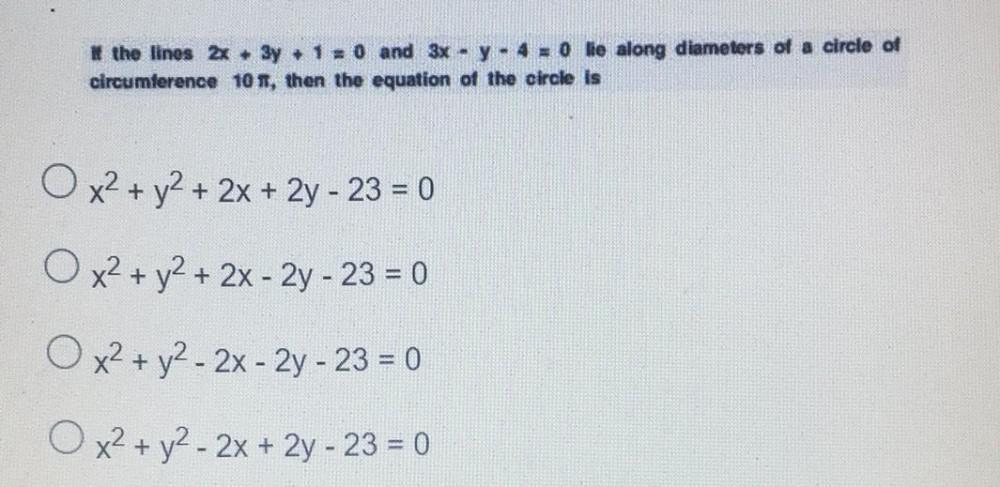Question:

# * the lines 2x + 3y + 1 = 0 and 3x - y - 4 = 0 lie along diameters of a circle of circumference 108, then the equation of the ci* the lines 2x + 3y + 1 = 0 and 3x - y - 4 = 0 lie along diameters of a circle of circumference 108, then the equation of the circle is O x2 + y2 + 2x + 2y - 23 = 0 O x2 + y2 + 2x - 2y - 23 = 0 O x2 + y2 - 2x - 2y - 23 = 0 O x2 + y2 - 2x + 2y - 23 = 0